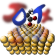JDFTx  1.7.0Q. How to restart an unfinished DFT calculation?

A. dump state with appropriate frequency.

Q. Why is initialization taking so long?

Q. Which is better for electronic steps, electronic-scf or electronic-minimize?

Q. How to speed up convergence?

A. For metallic systems, using elec-smearing helps

Q. What's the suggested parallelization scheme for most efficient calculation?

A. Dry run your calculation to find the number of k-points in the calculation (nStates), then set number of processes less than or equal to nStates, and as many threads as possible.

Q. Fillings mismatch error

A. Ensure that the number of bands (elec-n-bands) were not changed between subsequent calculations.

Q. Core overlapping error

A. Atoms are positioned too close to each other. Check units (Bohr by default) of ionic positions and lattice constants, and whether coords-type is set correctly. For example, if coords-type is set to lattice, then the ionic positions should conventionally be fractions.